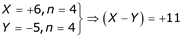# GATE Solved Paper 2017-19 - GATE 2019

• A

(x ⨁ y) ⨁ z = x ⨁ (y ⨁ z)• B

(x + y) ⨁ z = x ⨁ (y + z)• C

x ⨁ y = x + y, if xy = 0• D

x ⨁ y = (xy + x′y′)′• Option : B
• Explanation :
(a) x ⊕ y = (xy + x′y ′)′
= (xy )′
= x ⊕ y, it is valid.

(b) (x + y) ⊕ z = (x + y).z + (x + y)z
= xyz + xz + yz
= 1  4,6  2,6
= Σm(1, 2, 4, 6)
(x + y) ⊕ z = x(y+z) + x (y+z)
= xy + xz + yz
= 2,3  1,3  4
= Σm(1, 2, 3, 4)
(x + y) ⊕ z ≠ x ⊕ (y + z)
So option (b) is invalid.

(c) (x ⊕ y) ⊕ z = x ⊕ (y ⊕ z)
Associativity is true on Ex-OR operator so it valid.

(d) x ⊕ y = (x + y)(x + (y)
= (x + y)xy
= (x + y)x0
= (x + y), so it is valid.

• A

L⋅ LR {xy ⏐ x ∈ L, yR∈ L}• B

Suffix (L) = {y ∈ ∑* ⏐ ∃x ∈ ∑* such that xy ∈ L}• C

Prefix (L) = {x ∈ ∑* ⏐ ∃y ∈ ∑* such that xy ∈ L}• D

{wwR ⏐ w ∈ L}• Option : B
• Explanation :
If L is regular, L.LR is also regular by closure property.
Suffix (L) and Prefix (L) are also regular by closure property.
However option (b) {wwR |w∈L} need not be regular since if L is an infinite regular language, then {wwR |w ∈ L} will not only be infinite, but also non-regular. Since it involves string matching and we can increase in length indefinitely and then finite automata FA will run out of memory.

• A

n bits• B

n - 1 bits• C

n + 1 bits• D

n + 2 bits• Option : C
• Explanation :
For example:
Let,Hence,
Z = 11 which required 5 bits which is (n + 1) bits

• A

I implies II; II does not imply I.• B

II implies I; I does not imply II.• C

I does not imply II; II does not imply I.• D

I and II are equivalent statements.• Option : D
• Explanation :
Both I and II are equivalent statements.

• A

R1 and R2• B

R1 only• C

R2 only• D

Neither R1 nor R2• Option : B
• Explanation :
Given R1 is a equivalence relation, because it satisfied reflexive, symmetric, and transitive conditions:
• Reflexive: a = g–1ag can be satisfied by putting g = e, identity “e” always exists in a group.
• Symmetric:
aRb ⇒ a = g–1bg for some g
⇒ b = gag–1 = (g–1)–1ag–1
g–1 always exists for every g ∈ G.
• Transitive:
aRb and bRc ⇒ a = g1–1bg1
and b = g2–1 cg2 for some g1g2 ∈ G.
Now a = g1–1 g2–1 cg2g1 = (g2g1)–1 cg2g1
g1 ∈ G and g2 ∈ G ⇒ g2g1 ∈ G since group is closed so aRb and aRb ⇒ aRc
R2 is not equivalence because it does not satisfied reflexive condition of equivalence relation:
aR2a ⇒ a = a–1 ∀a which not be true in a group.
So, option (B) is correct.
Related Quiz.
GATE 2019